•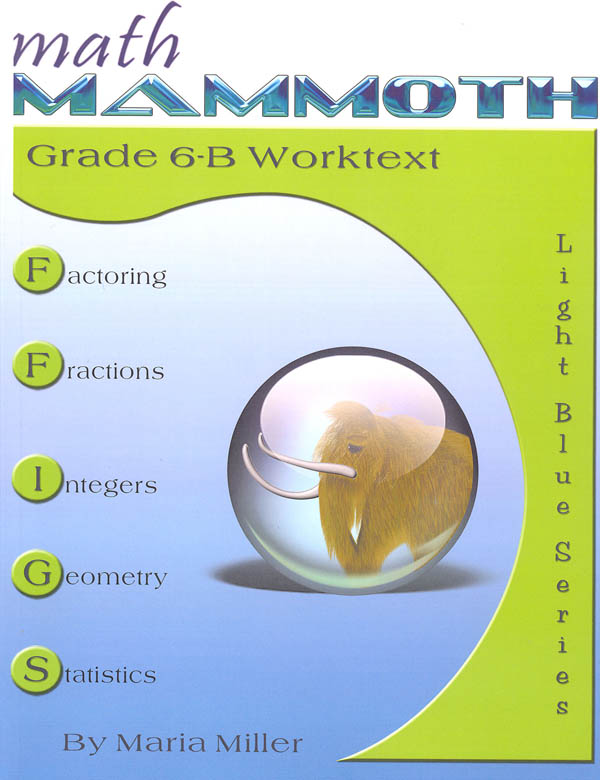•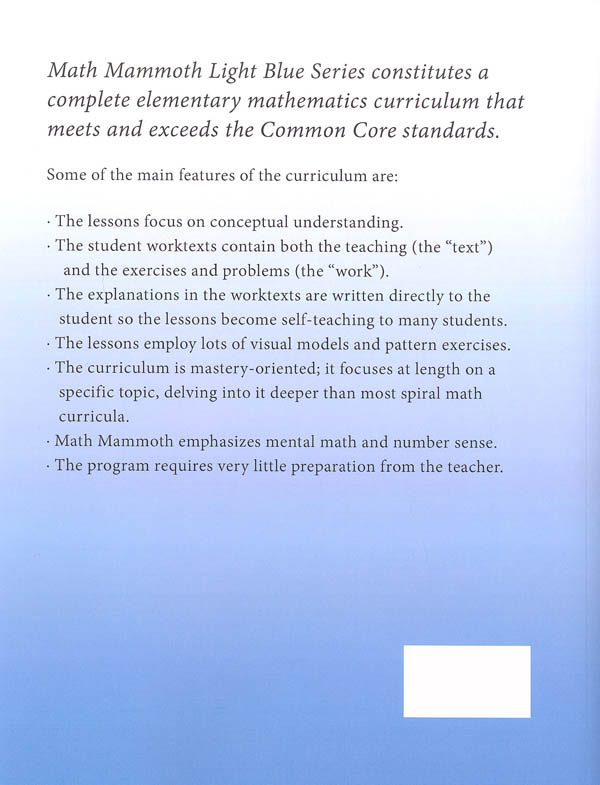•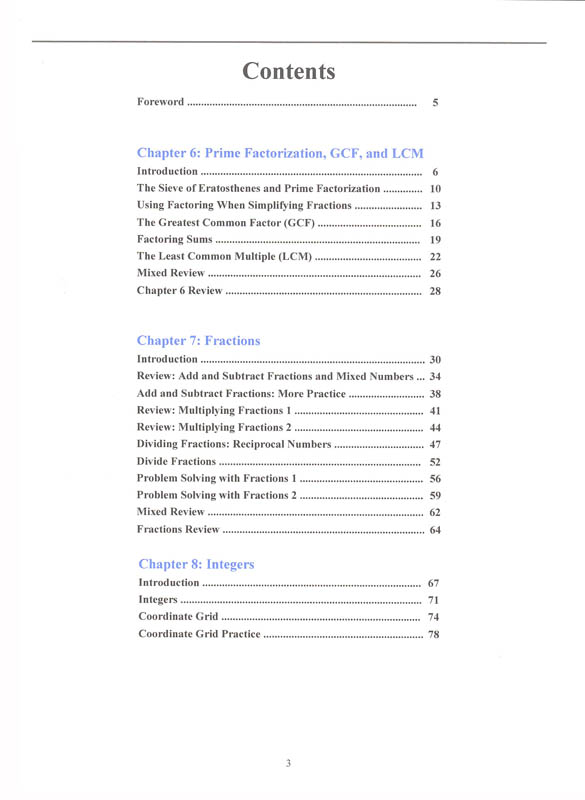•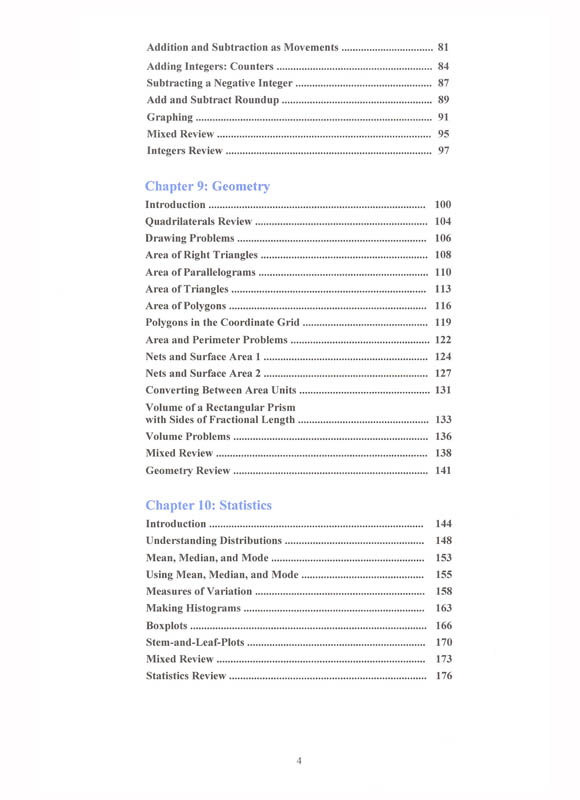•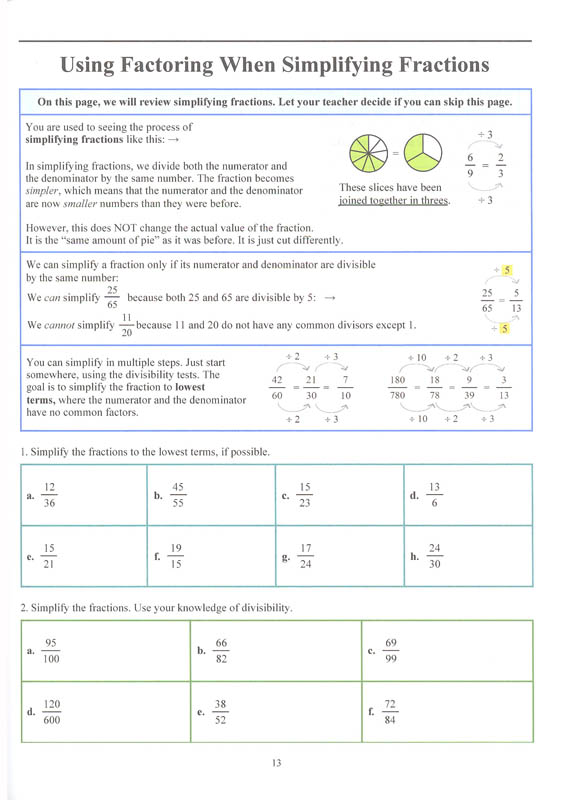•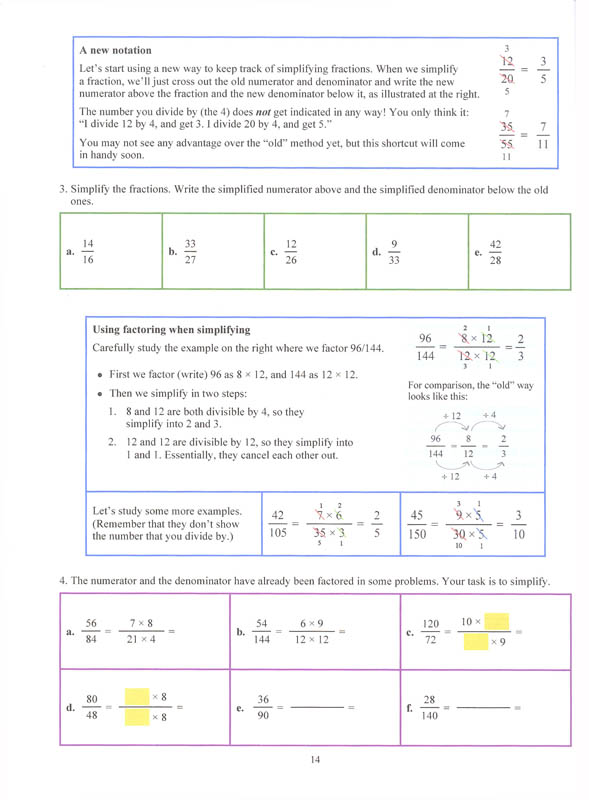•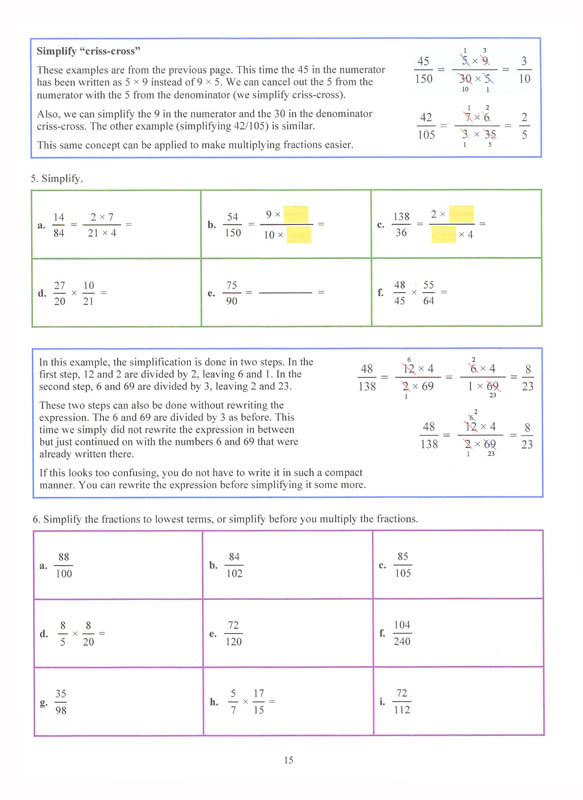# Math Mammoth Light Blue Series Grade 6-B Worktext (Colored Version)

# 062821

Our Price: \$17.75
Retail: \$21.45
Save: 17.25% (\$3.70)
In Stock.
Qty:
Qty:

 Item #: 062821 9781942715382 6

#### Publisher Description:

The two books (worktext part A and part B) deal with

• review of the basic operations with whole numbers
• beginning algebra topics: expressions, equations, and inequalities
• review of all decimal arithmetic
• introduction to ratios and percent
• prime factorization, GCF, and LCM
• a review of fraction arithmetic from 5th grade, plus a focus on division of fractions
• the concept of integers, coordinate grid, addition & subtraction of integers
• geometry: review of quadrilaterals & drawing problems; area of triangles & polygons; volume of rectangular prisms with fractional edge lengths; surface area
• statistics: concept of distribution, measures of center, measures of variation, boxplots, stem-and-leaf plots, histograms

In sixth grade, students encounter the beginnings of algebra, learning about algebraic expressions, one-variable equations and inequalities, integers, and ratios. We also review and deepen the students' understanding of rational numbers: both fractions and decimals are studied in depth, while percent is a new topic for 6th grade. In geometry, students learn to compute the area of various polygons, and also calculate volume and surface area of various solids. The last major area of study is statistics, where students learn to summarize and describe distributions using both measures of center and variability.

The year starts out with a review of the four operations with whole numbers (including long division), place value, and rounding. Students are also introduced to exponents and do some problem solving.

Chapter 2 starts the study of algebra topics, delving first into expressions and equations. Students practice writing expressions in many different ways, and use properties of operations and the idea of maintaining the equality of both sides of an equation to solve simple one-step equations. We also study briefly inequalities and using two variables.

Chapter 3 has to do with decimals. This is a long chapter, as we review all of decimal arithmetic, just using more decimal digits than in 5th grade. Students also convert measuring units in this chapter.

Ratios is a new topic (chapter 4). Students are already familiar with finding fractional parts from earlier grades, and now it is time to advance that knowledge into the study of ratios, which arise naturally from dividing a quantity into many equal parts. We study such topics as rates, unit rates, equivalent ratios, and problem solving using bar models.

Percent (chapter 5) is an important topic to understand thoroughly, because of it many applications in real life. The goal of this chapter is to develop a basic understanding of percent, to see percentages as decimals, and to learn to calculate discounts.

In chapter 6, we first review prime factorization, and then study the greatest common factor and the least common multiple.

Chapter 7 first presents a thorough review of fraction operations from 5th grade (addition, subtraction, and multiplication). The topic of focus in the chapter is fraction division. Students also do some problem solving with fractions.

In chapter 8, students are introduced to integers. They plot points in all four quadrants of the coordinate plane and learn to add and subtract with negative numbers. Multiplication and division of integers are studied in 7th grade.

In geometry (chapter 9), the focus is on the area of polygons. This is studied in a logical progression: first, the area of right triangles, then the area of parallelograms, then, the area of triangles, and lastly the area of polygons. We also review some topics from earlier grades (quadrilaterals, perimeter, basic drawing). Other major topics in this chapter are calculating surface area of some solids using nets, and volume of rectangular prisms with fractional edge lengths.

Lastly, in chapter 10, students study statistics. The focus idea is that of a statistical distribution. Students learn about measures of center and measures of variability. They learn how to make dot plots, histograms, boxplots, and stem-and-leaf plots. All of these lessons are necessary to summarize and analyze distributions.

Category Description for Math Mammoth:

Theres a whole lot to like about this well-conceived and inexpensive math program. The main features of this program, according to author Maria Miller, are:

• Focus is on conceptual understanding rather than rote problem-solving
• Teacher instructions are right in the book; no need to buy a separate teacher book
• Clear explanations are written for the student, so the lessons are virtually self-teaching
• Lessons use lots of visual and pattern exercises
• Mastery oriented:concentrates on each topic for a long time studying fewer topics per grade than with spiral
• Emphasis is on mental math and number sense
• Very little teacher preparation is required
• Content aligned to Common Core Standards

These are all valid claims and sum up this approach nicely. Its linear approach is similar to that of the MCP Math program. Topics are introduced, studied in depth, then the student proceeds to the next topic. Visually, it reminds me of Developmental Math because of the extensive use of pictorial representations. Users posting online have compared the course to Singapore Math, but I don't really see much similarity. While they both emphasize conceptual learning and mental computation skills, Math Mammoth has a much more traditional feel. A distinguishing feature of this program is the authors amazing ability to simplify and clarify math processes so children can understand them easily. Even in printed form, this course is the easiest on the budget using a strictly by-grade comparison. And for those purchasing the complete program on CD (or download), the savings are even greater. Imagine getting a complete, masterfully taught math curriculum for grades 1-7 for less than \$150. I would call that frugal and smart.

Student Workbooks A and B for each level have varying numbers of chapters, each focusing on a math topic. These are sometimes very broad. For example, one very large chapter (68 pages!) in Worktext 4-B is simply titled, “Division”. It reviews division, and then covers every facet of fourth grade division. Since division skills are being built step-by-step throughout the chapter, there’s really no need for review or testing until the end; but it does make for a long teaching unit. Each chapter begins with a short introduction. This is where you will find any teacher instruction for the chapter, including background information, strategies and ideas for teaching lesson concepts, a summary of lessons by title (including number of pages for each), and a list of Helpful Internet Resources for further exploration, practice and enrichment. If the chapter is on a skill introduced in a previous grade, the skill is reviewed before the new material is introduced. Lessons each include a complete explanation of each skill being taught, numerous examples and models (many visual) to help students understand the concepts behind the math, and a reasonable number of problems for students to work to ensure comprehension.

The lessons vary from 1-5 pages each. For planning, the author suggests simply dividing the number of pages in each book by the teaching days rather than planning to teach a lesson a day. This would generally mean covering 1-2 pages a day depending on grade level. After the last lesson in each chapter there are one or two reviews. Chapter tests are contained in the separate Tests and Cumulative Reviews Book, as are cumulative reviews (taken upon completion of each worktext chapter) and a comprehensive test for the grade level. A nice feature is the inclusion of grading instructions and scales. If remediation is indicated, the author provides a website (www. homeschoolmath.net) for additional math worksheets by grade level and skill. Worksheets are randomly generated within your provided parameters, so each is different.

Use the Skills Review Workbooks books alongside the Math Mammoth curriculum to review concepts that are being taught. Each book is divided into chapters that correspond with the curriculum chapters. These workbooks are optional, and are intended for those who want a spiral review. Each review in the workbook is one page in length and includes a variety of math exercises. Students might write math equations, color pictures, or solve puzzles to put their math skills to the test. Some children require more practice than others, so use only the review pages you need. Skills Review Workbooks are printed with color for a little more visual appeal, and the number of reviews vary from book to book. Answer Keys are a separate book and are also printed in color.

As mentioned briefly before, I find the author’s teaching methods very solid, efficient, and effective. Her goal is to imbue the student with an understanding of the concepts in math rather than just how to solve problems and this is evident in her instruction. She consistently shares strategies, “tricks” and revealing insights into the mysteries of math, essentially putting a teacher right on the page. An example is in teaching beginners to add numbers with sums greater than 10. She tells students that when adding 9 to a number, the 9 “really wants to be a ten”, so you should take one from the other number to make it a ten, and then add the rest. I have seen few other curriculums (see Miquon Math) that teach students alternate, flexible ways like this to approach operations. Another feature I liked in the book was using gridded workspaces for solving problems. Especially for students just starting to use multiple-digit operations, it’s helpful to get them in the habit of keeping answers neatly aligned in columns. I also like the scale problems in Grade 4’s multiplication and other chapters. These are much like the ones in CTP’s Balance Math program and very effective for understanding how equations work. There’s also a healthy balance of word problems in the lessons with realistic, practical contexts. The geometry sections are particularly good, too, with ample hands-on work. The author even includes printable manipulatives for constructing your own geometric solids.

Category Description for COMPREHENSIVE PROGRAMS - ELEMENTARY:

Primary Subject
Mathematics
6
ISBN
9781942715382
Format
Binder
Brand Name
Taina Maria Miller
Weight
1.3 (lbs.)
Dimensions
11.06" x 8.56" x 0.5"
Browse 1 question Browse 1 question and 9 answers
Why did you choose this?
Rainbow Resource Center Store
excellent math curriculum
on Jan 19, 2021
I really enjoy Math Mammoth's approach to math and it has worked really well with my right brained child.
on Jul 8, 2020
excellent math curriculum
on Jan 19, 2021
We enjoyed using the Grade 5 Math Mammoth books last year!
on Aug 27, 2020
We enjoyed using the Grade 5 Math Mammoth books last year!
on Aug 27, 2020
We have been using this curriculum for all four of our kids. It is thorough with multiple approaches per concept.
on May 28, 2020
There are no reviews for this item. Write Review

help desk software